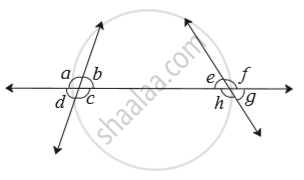# Observe the Angles Shown in the Figure and Write the Following Pair of Angle. Corresponding Angles - Mathematics

Sum

Observe the angles shown in the figure and write the following pair of angle.Corresponding angles

#### Solution

Corresponding angles :  If the arms on the transversal of a pair of angles are in the same direction and the other arms are on the same side of  transversal, then it is called a pair of corresponding angles.

Corresponding angles
(a) ∠d and ∠h
(b) ∠c and ∠g
(c) ∠a and ∠e
(d) ∠b and ∠f

Concept: Pairs of Lines - Transversal
Is there an error in this question or solution?

#### APPEARS IN

Balbharati Mathematics 8th Standard Maharashtra State Board
Chapter 2 Parallel lines and transversals
Practice Set 2.1 | Q 1.2 | Page 8